### SIN Function Examples – Excel, VBA, & Google Sheets

This tutorial demonstrates how to use the Excel SIN Function in Excel to calculate the sine.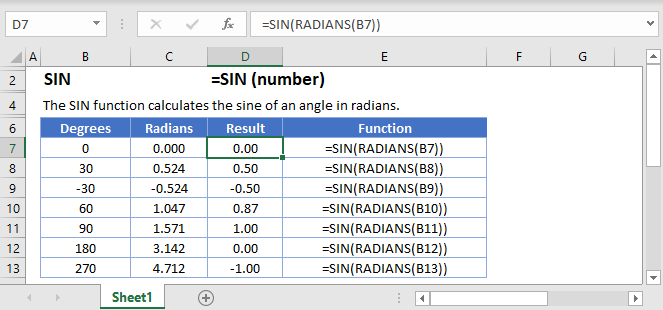## SIN Function Overview

The SIN Function Returns the sine of an angle.

To use the SIN Excel Worksheet Function, select a cell and type: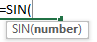(Notice how the formula inputs appear)

### SIN Function Syntax and Inputs:

`=SIN(number)`

number – A number.

AutoMacro - VBA Code Generator

### Degrees and Radians

The input number for the SIN function is an angle in radians. If we want to use degrees, we must first convert from degrees into radians. This can be done in a couple of ways:

#### Method 1: Use math knowledge

To convert from degrees to radians, we multiply our number (in degrees) by π/180. For example, if we want to know the sine of 45° we could use the formula

`=SIN(45 * PI() / 180)`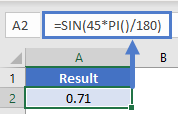#### Method 2: Excel’s inbuilt conversion function

Luckily, we don’t need to look up or remember how to convert to radians from degrees. Instead we could just use another Excel function: RADIANS. This function converts a number from degrees into radians. Again, if we want to know the sine of 45° we could use

`=SIN(RADIANS(45))`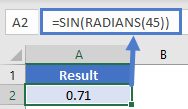Each of these methods is shown in the image below.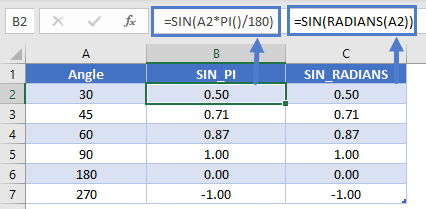### Creating a sine curve

To create a sine curve in Excel, we need to first choose our start and end points and then list out a lot of numbers. Let’s go from -2π up to 2π in increments of 0.1.

`=SIN(C3)`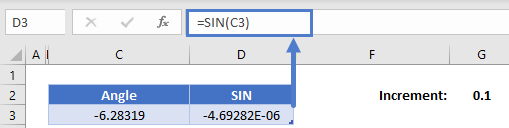Next, we’re going to add the 0.1 onto the angle and then calculate the sine of that angle. Use the formula

`=C3+\$G\$2`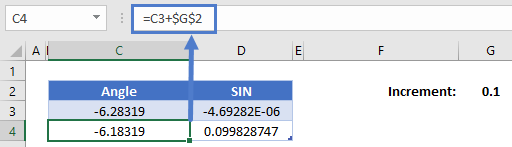(the \$ signs lock G2 so the formula will always reference that 0.1 even if we copy the formula!)

Now highlight both the new angle and sine function that we’ve calculated, hold the handle, and drag it down until our angle reaches about 2π (about 6.28)

Highlight the entire range of both angles and sines, click insert, find the graphs and select “Scatter with Smooth Lines”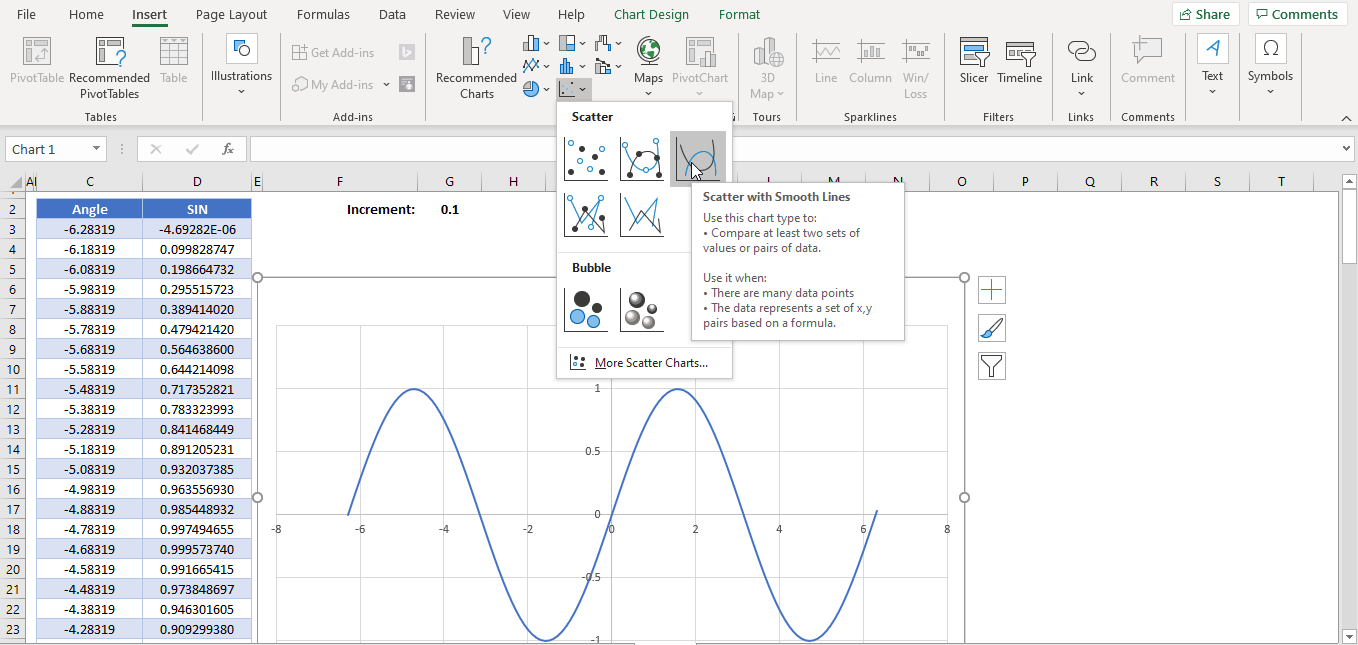#### Common Errors

#NAME? This error might occur if the function is not written correctly (e.g., SINE(45) instead of SIN(45)) or if a character other than a number has been entered into the argument (e.g., SIN(x)). To correct this, make sure you function is spelled correctly and the argument is a number.

#VALUE! This error might occur a range has been entered for the argument of the function (e.g., SIN(C5:C7)). To correct this, keep in mind that only one value can bentered for the SIN function.

## SIN in Google Sheets

The SIN Function works exactly the same in Google Sheets as in Excel: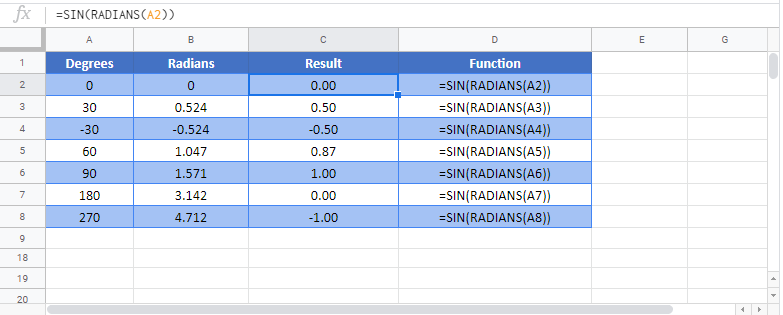## SIN Examples in VBA

You can also use the SIN function in VBA. Type:

```Dim val1 as double
val1 = sin(number)```

For the function arguments (number, etc.), you can either enter them directly into the function, or define variables to use as we have done here.

### Common Errors in VBA

Run-time error ’13’: Type mismatch This error occurs when a non-number has been entered into the argument.

## Excel Practice Worksheet

Practice Excel functions and formulas with our 100% free practice worksheets!

• Automatically Graded Exercises
• Learn Excel, Inside Excel!